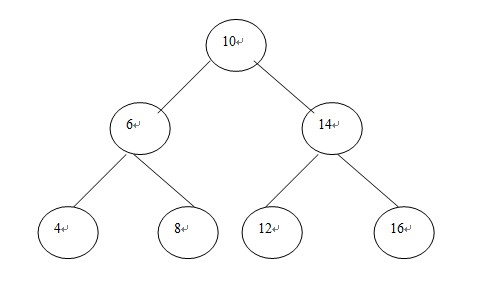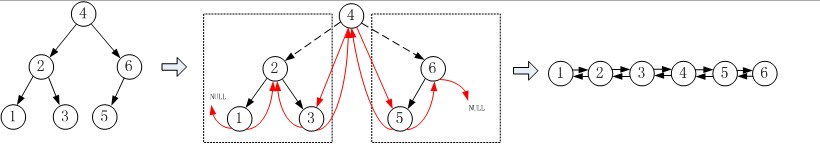# 关于二叉查找树转换成双向排序链表

Jan 20, 2013

1、设x为二叉树中的一个节点，在这个节点上，如果存在左子树，则其左子树每一个节点的值只会小于或者等于x
2、设x为二叉树中的一个节点，在这个节点上，如果存在右子树，则其右子树每一个节点的值只会大于或者等于x

1、中序遍历：某一树的根在左子树与右子树之间输出
2、前序遍历：某一树的根在左右子树之前输出
3、后续遍历：某一树的根在左右子树之后输出

1、某一个节点x的后继就是大于x的关键字中最小的那个节点，前驱就是小于x的关键字中最大的那个节点。当该节点有左子树时，只要遍历左子树找到左子树中最大的一个节点即可。如无左子树，则指针不停的向上移动(设x是当前节点)，`node *y = x->parent`，如此遍历直到x是y的右子树为止。因为如果是左子树，父节点只能位于该节点的后边，因此不可能是前驱节点。
2、查找后继节点，如果该节点有右子树，则在其有子树中查找最小的那个节点即为后继节点。如果节点没有右子树。与查找前驱类似，指针不停的向上移动(设x为当前节点，即`node *y = x->parent`),直到x是y的左子树为止，因为如果是右子树，父节点只能位于该节点的前边，不可能是后继节点。

``````typedef struct Node
{
int KeyValue;
struct Node* p_left_child_node;
struct Node* p_right_child_node;
struct Node* p_father_node;
}Node,*PNode;
``````

``````void create_BST_tree(PNode* root,int key_array[],int node_number)
{
//逐个结点插入二叉树中
for(int i = 0;i < node_number; i++)
insert_node(root,key_array[i]);
}
``````

``````//有可能会改变节点指针，所以用二级指针，当然也可以指针引用
void insert_node(PNode* p_root,int KeyValue)
{
PNode p_node = (PNode)malloc(sizeof(Node));
p_node->KeyValue = KeyValue;
p_node->p_left_child_node = NULL;
p_node->p_right_child_node = NULL;
p_node->p_father_node = NULL;

if((*p_root) == NULL)
{
*p_root = p_node;
return ;
}
if((*p_root)->p_left_child_node == NULL && (*p_root)->KeyValue > KeyValue)
{
p_node->p_father_node = (*p_root);
(*p_root)->p_left_child_node = p_node;
return ;
}
if((*p_root)->p_right_child_node == NULL && (*p_root)->KeyValue < KeyValue)
{
p_node->p_father_node = (*p_root);
(*p_root)->p_right_child_node = p_node;
return ;
}
//递归方式
if((*p_root)->KeyValue > KeyValue)
insert_node(&(*p_root)->p_left_child_node,KeyValue);
else
insert_node(&(*p_root)->p_right_child_node,KeyValue);
return ;
}
``````

``````PNode search(PNode p_root,int KeyValue)
{
if(p_root->KeyValue > KeyValue)
return search(p_root->p_left_child_node,KeyValue);
else if(p_root->KeyValue < KeyValue)
return search(p_root->p_right_child_node,KeyValue);
return p_root;
}
``````

``````PNode search_MAX(PNode p_root)
{
if(p_root == NULL)
return NULL;
if(p_root->p_right_child_node == NULL)
return p_root;
else
return search_MAX(p_root->p_right_child_node);
}
``````

``````PNode search_MIN(PNode p_root)
{
if(p_root == NULL)
return NULL;
if(p_root->p_left_child_node == NULL)
return p_root;
else
return search_MIN(p_root->p_left_child_node);
}
``````

``````PNode search_precursor_node(PNode p_node)
{
if(p_node == NULL)
return NULL;
//如果说该节点有左子树，则在左子树中一直找，直到找到最大的节点就返回
if(p_node->p_left_child_node)
return search_MAX(p_node->p_left_child_node);
else
{
PNode y = p_node->p_father_node;
//如果是没有左子树
if(p_node->p_father_node == NULL)
return NULL;
else
{
while(y != NULL && p_node == y->p_left_child_node)
{
p_node = y;
y = y->p_father_node;
}
}
return y;
}
}
``````

``````PNode search_succeed_node(PNode p_node)
{
if (p_node == NULL)
{
return NULL;
}
if (p_node->p_right_child_node)
{
search_MIN(p_node->p_right_child_node);
}
else
{
PNode y = p_node->p_father_node;
//如果是没有左子树
if(p_node->p_father_node == NULL)
return NULL;
else
{
while(y != NULL && p_node == y->p_right_child_node)
{
p_node = y;
y = y->p_father_node;
}
}
return y;
}
}
``````

``````int _tmain(int argc, _TCHAR* argv[])
{
PNode root=NULL;
int nodeArray={15,6,18,3,7,17,20,2,4,13,9};
create_BST_tree(&root,nodeArray,11);
cout << search(root,20)->KeyValue << endl;
cout << search_MAX(root)->KeyValue << endl;
cout << search_MIN(root)->KeyValue << endl;
cout << search_precursor_node(search(root,18))->KeyValue << endl;
cout << search_succeed_node(search(root,20))->KeyValue << endl;
return 0;
}
``````（此图转自网络，只为说明一下转换方法)

``````typedef struct TNode
{
int value;
TNode* lchild;
TNode* rchild;
}TNode,*BTree;

//二叉树转换为双向链表
TNode* TreeToList(BTree tree,TNode* &lastNode)
{
TNode* head;
//若树为空，返回空
if (tree == NULL)
{
lastNode = NULL;
return NULL;
}
//若无左子树，则该根节点为链表的头结点
if (tree->lchild==NULL)
{
head = tree;
}
//若有左子树，递归调用转换函数将左子树转换为双向链表
//左子树转换所得链表的头结点是整个树的头结点
//左子树链表的尾结点与根节点相邻
else
{
head = TreeToList(tree->lchild,lastNode);
tree->lchild = lastNode;
tree->lchild = lastNode;
if( (*lastNode) != nullptr )
{
lastNode->rchild = tree;
}
}
//若无右子树，则该根节点为链表的尾结点
if (tree->rchild==NULL)
{
lastNode = tree;

}
//若有右子树，递归调用转换函数将左子树转换为双向链表
//右子树转换所得链表的尾结点是整个树的尾结点
//右子树链表的头结点与根节点相邻
else
{
tree->rchild = TreeToList(tree->rchild,lastNode);
tree->rchild->lchild = tree;
}
return head;
}
``````